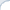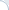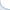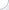Eight years ago I started my blog with a post on the origins of dynamic programming. Therein, I argue that the term programming stems from a military definition of the word "program", which simply means planning and logistics. In mathematics, this term was adopted to denote optimization problems and gave rise to several names such as integer, convex, non-linear, and differentiable programming. I promised to describe how dynamic programming had a somewhat rocky start in computational biology in a follow-up post, but never delivered on this promise.

It has been a decade of phenomenal success of another programming concept, namely the differential programming. Three neural networks pioneers: Geoffrey Hinton, Yoshua Bengio, and Yann LeCun (with a regretful omission of Jürgen Schmidhuber) received a Turing award for "For conceptual and engineering breakthroughs that have made deep neural networks a critical component of computing." Now it seems to be perfect time to deliver on the promise and wrap up with historical dynamic programming posts.

As I mentioned in my first blog post, dynamic programming is a relatively simple way to solve complex problems through an elegant and efficient recursion. In particular, it is at the heart of evolutionary distances in computational biology and the Levenshtein (also known as an edit) distance in natural language processing. Different as they are, these fields rely on string comparison via variants of an edit distance. The simplest way to compute an unweighted edit distance between strings $a=a_1 a_2 \ldots a_n$ and $b = b_1 b_2 \ldots b_m$ is through a following simple recursion:

$$d_{i+1,j+1} = \min \left\{ \begin{array}{c} d_{i,j+1} + 1 \\ d_{i+1,j} + 1 \\ d_{i,j} + (a_{i+1} \ne b_{j+1})\\ \end{array} \right.$$

The computational cost is quadratic. Although there are faster average-case algorithms, there is little hope that the worst-case time is strongly sub-quadratic.

The formula is simple, but not always immediately obvious. In fact, it took the scientific community almost ten years to fully realize that essentially the same approach provides a solution for several fields: information retrieval, bioinformatics, and speech recognition (see my survey for a list of early works). Furthermore, as I explain below, a renowned mathematician Stanislaw Ulam not only failed to discover the formula, but also failed to recognize the solution when it was presented to him by David Sankoff. Next time when you fail to solve an apparently "simple" dynamic programming puzzle, do not beat yourself up!

The edit distance was first published by Levenshtein (and is often called the Levenshtein distance) in the context of self-correcting binary codes (Levenshtein, Vladimir I. "Binary codes capable of correcting deletions, insertions, and reversals." Soviet physics doklady. Vol. 10. No. 8. 1966.). Imagine a noisy channel that modifies binary messages by changing, inserting, and deleting bits occasionally. If such spurious changes are not very frequent, it may still be possible to recover the original message. This is clearly possible but only if the code of one symbol is sufficiently different from other symbol codes (i.e., when the distance is large enough).

Consider an example, where the set of codes contains only two 3-bit codes 000 and 111 and the channel cannot modify more than one bit. Then, a noisy version of 000 will always be different from the noisy version of the code 111. Indeed, the noisy version of 000 can have at most one bit equal to one, while the noisy version of 111 always has at least two bits equal to one. By counting the number of unit bits, we can always recover the original code.

Clearly, there is a lot of waste here, because we use only two values out of eight possible ones. However, this is the price we pay to separate the noisy variants of the codes. Levenstein himself was only interested in estimating the amount of waste that is necessary to make the garbled codes separable. He actually proved that the waste would not be that bad: It is possible to use about $2^n/n$ separable code words of length n (bits).

Despite Levenshtein paper is massively cited, I think few people read it, in particular, because it was not possible to access this paper online. For my survey on approximate dictionary searching, I had to visit the actual Russian library to read the paper. It was a bit complicated, because at that time I already resided in the US.

Levenstein apparently did not realize that the distance he introduced could be useful in text processing applications, such as spell-checking, speech recognition, and alignment of DNA (or protein) sequences. In the 60s, the bioinformatics was in its early stage. The structure of the DNA was discovered in 1953, but the first complete gene was not decoded until 1972! Nevertheless, there was apparently a lot of interest in the problem of sequencing and finding similar regions among sequences of different species (note, however, this is not the only goal of finding similar sequences).

The usefulness of the latter method is based on the assumption that similarities in genetic sequences represent common ancestry. Two species start from a common genome and then follow different evolutionary paths, which results in changing certain areas of the original DNA. Yet, most subsequences of the DNA remain very similar. In a simplified model, the Nature "edits" a sequence by randomly deleting, inserting, or modifying certain small areas of the genes. These changes happen with a certain rate: The longer is a time frame, the more changes happen. By measuring the volume of the differences between two species (using some form of the edit distance) we can estimate the time required to evolve from a single common ancestor. (See, e.g., Nei, M., & Zhang, J. (2006). Evolutionary Distance: Estimation for more details.)

Stanislaw Ulam, a famous mathematician who played one of the pivotal roles in the Manhattan project, is often credited with the invention of the evolutionary distance. However, as argued by David Sankoff, he failed to realize that the distance can be computed by a simple dynamic programming algorithm. Turns out that dynamic programming was not so simple after all.